top of page• # GATE Previous Year Questions

Transpose of a Matrix:

Matrix formed by exchanging rows and corresponding columns of the original matrix. Transpose of a matrix A:

Transpose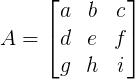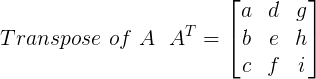# * Value of determinant of [A] and transpose of [A] is same i.e. lAl = lTrans. Al

Null Matrix:

Matrix having all the elements as zero.

Null Mat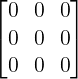This matrix transforms all vectors in the space to zero vector i.e. at the origin.

Square Matrix:

Matrix having equal number of rows and columns. A 2 x 2 matrix is:

Sqr Mat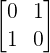2 x 2 square matrix transforms a 2-D vector such that it remains in 2-D plane only. Likewise, a 3 x 3 square matrix transforms a 3-D vector such that remains in 3-D space only. Whereas a non-square matrix may transform a 2-D vector into 3-D vector and vice-versa.

Diagonal Matrix:

A square matrix having only non-zero diagonal elements. All other elements of the matrix are zero.

diag matA diagonal matrix is called 'Scalar Matrix' when a = b. A scalar matrix only stretches or shrinks a vector along its span.

A diagonal matrix is called a 'Unit/Identity Matrix' if a = b = 1. Unit matrix is represented by I and does nothing to the vector after transformation. A 3x3 unit matrix is:

scala mat
uni mat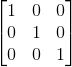​​Symmetric and Skew-Symmetric Matrix:

A square matrix is called 'Symmetric' if

(a)ij  = (a)ji

Hence; Trans.[A] = [A]

A 3 x 3 symmetric matrix is:

sym skew matFor a 'Skew-Symmetric Matrix'

(a)ij  = -(a)ji and (a)ii = 0

Hence;Trans.[A] = -[A]

A 3 x 3 skew-symmetric matrix is: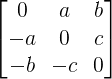Orthogonal Matrix:

A square matrix satisfying the condition

ortho matAn orthogonal matrix transforms a space such that axes always remains perpendicular to each other and magnitude of unit vectors remains unchanged (rotation/reflection). Consider an orthogonal matrix:For the above matrix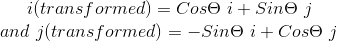so i(transformed) and j(transformed) are perpendicular to each other and their magnitude is equal to 1.

* Value of determinant of an orthogonal matrix is equal to 1 or -1 i.e. lAl = 1 or -1.

Idempotent Matrix:

For an idempotent matrix

idem matNilpotent Matrix:

​For a nilpotent matrix

nilpo matk is a positive integer.

Least positive integer for which above value is true is called index of the matrix.

Involuntary Matrix:

For an involuntary matrix

invol matIt means matrix is inverse of itself.

​​Singular Matrix:

A matrix is called a singular matrix if its determinant is zero i.e. lAl = 0.

​Triangular Matrix:

A square matrix having all elements below the main diagonal as zero is called 'Upper Triangular (UT) Matrix'. For upper triangular matrix (a)ij = 0 for all i>j.

sing mat
traing matA square matrix having all elements above the main diagonal as zero is called 'Lower Triangular (LT) Matrix'. For lower triangular matrix (a)ij = 0 for all i<j.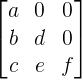* Transpose of a LT Matrix is a UT matrix.

* Determinant of triangular matrix is equal to product of diagonal elements.

Complex Matrices:

Matrix whose elements may be complex numbers.

a) Conjugate of a Matrix:

Matrix formed by conjugates of complex elements of original matrix.

# ​

Comp mat# A complex square matrix is called hermitian if (a)ij  = conjugate of (a)ji. A 2 x 2 Hermitian matrix is:# A complex square matrix is called Skew-Hermitian if (a)ij = - conjugate of (a)ji. A 2 x 2 Skew-Hermitian matrix is:# GATE 2016: A real square matrix A is called skew-symmetric if

Gate ques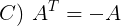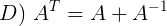# GATE 2009: For a Matrix [M] as given below, the transpose of the matrix is equal to the inverse of the matrix. The value of x is:# GATE 2006: Multiplication of matrices E & F is G. Matrix E and G are as given below. What is the matrix F?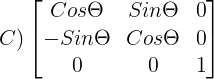# GATE 2004: For which value of x, the matrix given below will become singular: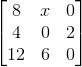# D) All eigenvalue of P are real numbers.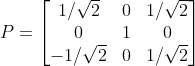bottom of page